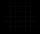# One half

One half ofis:

« Correct result#### Solution:Leave us a comment of example and its solution (i.e. if it is still somewhat unclear...):Be the first to comment!## Next similar examples:

1. PowersExpress the expression ? as the n-th power of the base 10.
2. PowerNumber ?. Find the value of x.
3. EquationHow many real roots has equation ? ?
4. Is equalIs equal following terms? ?
5. TreesA certain species of tree grows an average of 0.5 cm per week. Write an equation for the sequence that represents the weekly height of this tree in centimeters if the measurements begin when the tree is 200 centimeters tall.
6. 6 termsFind the first six terms of the sequence. a1 = 7, an = an-1 + 6
7. Nineteenth memberFind the nineteenth member of the arithmetic sequence: a1=33 d=5 find a19
8. AP - simpleDetermine the first nine elements of sequence if a10 = -1 and d = 4
9. First manWhat is the likelihood of a random event where are five men and seven women first will leave the man?
10. Factory and divisionsThe factory consists of three auxiliary divisions total 2,406 employees. The second division has 76 employees less than 1st division and 3rd division has 212 employees more than the 2nd. How many employees has each division?
11. Volleyball8 girls wants to play volleyball against boys. On the field at one time can be six players per team. How many initial teams of this girls may trainer to choose?
12. Two equationsSolve equations (use adding and subtracting of linear equations): -4x+11y=5 6x-11y=-5
13. Functions f,gFind g(1) if g(x) = 3x - x2 Find f(5) if f(x) = x + 1/2
14. Linear systemSolve a set of two equations of two unknowns: 1.5x+1.2y=0.6 0.8x-0.2y=2
15. Three workshopsThere are 2743 people working in three workshops. In the second workshop works 140 people more than in the first and in third works 4.2 times more than the second one. How many people work in each workshop?
16. The ballThe ball was discounted by 10 percent and then again by 30 percent. How many percent of the original price is now?
17. Theorem proveWe want to prove the sentence: If the natural number n is divisible by six, then n is divisible by three. From what assumption we started?